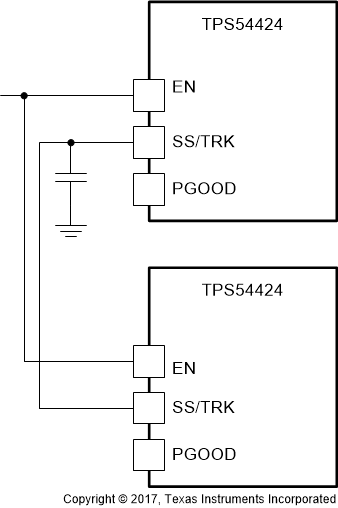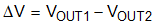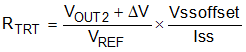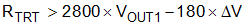SLVSDV8 July   2017

PRODUCTION DATA.

1. Features
2. Applications
3. Description
4. Revision History
5. Pin Configuration and Functions
6. Specifications
7. Detailed Description
1. 7.1 Overview
2. 7.2 Functional Block Diagram
3. 7.3 Feature Description
4. 7.4 Device Functional Modes
8. Application and Implementation
1. 8.1 Application Information
2. 8.2 Typical Application
1. 8.2.1 Design Requirements
2. 8.2.2 Detailed Design Procedure
3. 8.2.3 Application Curves
9. Power Supply Recommendations
10. 10Layout
11. 11Device and Documentation Support
12. 12Mechanical, Packaging, and Orderable Information

#### Package Options

Refer to the PDF data sheet for device specific package drawings

• RNV|18
• RNV|18

#### 7.3.10 Sequencing (SS/TRK)

Many of the common power supply sequencing methods can be implemented using the SS/TRK, EN and PGOOD pins.

The sequential method is illustrated in Figure 28 using two TPS54424 or similar devices. The power good of the first device is coupled to the EN pin of the second device which enables the second power supply once the primary supply reaches regulation.

Figure 29 shows the method implementing ratiometric sequencing by connecting the SS/TRK pins of two devices together. The regulator outputs ramp up and reach regulation at the same time. When calculating the soft-start time the current source must be doubled in Equation 4.Figure 28. Sequential Start-Up SequenceFigure 29. Ratiometric Start-Up Sequence

Ratiometric and simultaneous power supply sequencing can be implemented by connecting the resistor network of RTRT and RTRB shown in Figure 30 to the output of the power supply that needs to be tracked or another voltage reference source. Using Equation 6 and Equation 7, the tracking resistors can be calculated to initiate the VOUT2 slightly before, after or at the same time as VOUT1. Equation 5 is the voltage difference between VOUT1 and VOUT2.

To design a ratiometric start-up in which the VOUT2 voltage is slightly greater than the VOUT1 voltage when VOUT2 reaches regulation, use a negative number in Equation 6 and Equation 7 for deltaV. Equation 5 results in a positive number for applications where the VOUT2 is slightly lower than VOUT1 when VOUT2 regulation is achieved.

The deltaV variable is zero volts for simultaneous sequencing. To minimize the effect of the inherent SS/TRK to FB offset (Vssoffset = 25 mV) in the soft-start circuit and the offset created by the pull-up current source (Iss = 5 μA) and tracking resistors, the Vssoffset and Iss are included as variables in the equations.

To ensure proper operation of the device, the calculated RTRT value from Equation 6 must be greater than the value calculated in Equation 8.

Equation 5.vertical spacer

Equation 6.vertical spacer

Equation 7.vertical spacer

Equation 8.Figure 30. Ratiometric and Simultaneous Start-Up Sequence